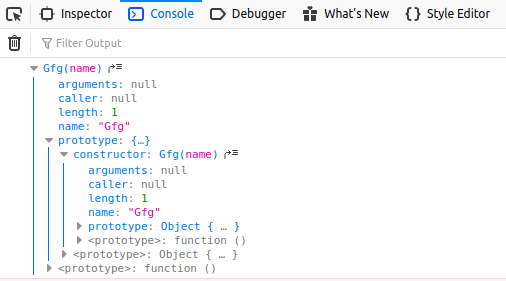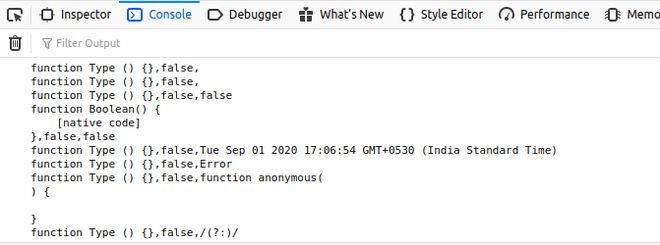Open in App
Not now

# JavaScript Object.prototype.constructor Property

• Last Updated : 29 Jun, 2022

The constructor property returns a reference to the object constructor function that has created the instance of an object. The value of the constructor is not a string containing the function’s name, but it is a reference to the function itself.

Syntax:

`Object.constructor`

Return Value: It is a reference to the object of constructor.

Example 1: Below example illustrates how to display the constructor of an object.

## HTML

 ```<``html``>` `<``body``>``    ``<``script` `type``=``"text/javascript"``>``        ``function Gfg(name) {``            ``this.name = name``        ``}``        ``let GeeksforGeeks = new Gfg('Geeks');``        ``console.log(GeeksforGeeks.constructor);``    ````` ``

Output:Example 2: Below example illustrates how to change the constructor of an object.

## HTML

 ```<``html``>` `<``body``>``    ``<``script` `type``=``"text/javascript"``>``        ``function Types() { }``        ``let types = [``            ``new Array(),``            ``[],``            ``new Boolean(),``            ``false,``            ``new Date(),``            ``new Error(),``            ``new Function(),``            ``new RegExp(),``            ``/(?:)/``        ``]``        ``let j = 0;``        ``while (j < ``types.length``) {``            ``types[j]``.constructor` `= ``Types``            ``types[j] = [types[j].constructor,``            ``types[j] instanceof Types,``            ``types[j].toString()]``            ``++j;``        ``}``        ``console.log(types.join('\n'));``    ````` ``

Output:Browser supported:

• Chrome 1 and above
• Edge 12 and above
• Firefox 1 and above
• Internet Explorer 11 and above
• Opera 9.5 and above
• Safari 3 and above

My Personal Notes arrow_drop_up